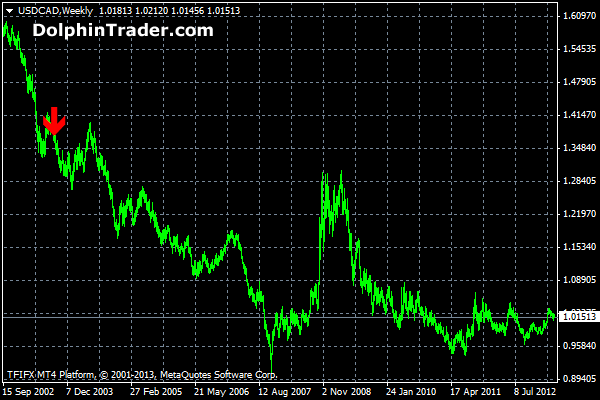# Smoothed Moving Average Crossover Signals Metatrader 4 Indicator

The Smoothed Moving Average (SMMA) Crossover Signals indicator is a trend following indicator based on the crossover of two smoothed moving averages. You can fully modify FastSMMA and SlowSMMA input values. Note: Smoothed Moving Averages give most recent currency prices an equal weighting to its historical prices.

Formule SMMA

SUM1 = SUM(CLOSE, N)
SMMA1 = SUM1/N

PREVSUM = SMMA(i-1) *N
SMMA(i) = (PREVSUM-SMMA(i-1)+CLOSE(i))/N